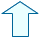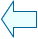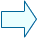The simplification of fractions Fractions

1. The simplification of a fraction.

All the fractions equivalent among them represent the same rational number. Therefore, in order to express the same value you should use the easiest fraction (= the fraction with the smallest numerator and denominator)
This fraction is called the reduced fraction, the fraction in its lowest terms or in its simplest form, because it cannot be simplified any further.
We apply the main property of division. We know that if we multiply or divide the numerator and the denominator by the same number, we will obtain another equivalent fraction.

In order to simplify a fraction, we have to find a number that can be both the divisor of the numerator and the denominator. Divide both of them by this number.

We should divide them by the largest possible number, that number is the highest common factor of both. In this way, we will get to the fraction in its lower terms in one go.

 In this window a random fraction appears, it also shows the common factors of the numerator and of the denominator. - Type the number below. You can divide the numerator and denominator by the typed number to simplify this fraction.Eduardo Barbero CorralSpanish Ministry of Education. Year 2007